• Machine Learning
• Urvashi Jaitley
• FEB 08, 2019

# Comparing Different Classification Machine Learning Models for an imbalanced dataset

A data set is called imbalanced if it contains many more samples from one class than from the rest of the classes. Data sets are unbalanced when at least one class is represented by only a small number of training examples (called the minority class) while other classes make up the majority. In this scenario, classifiers can have good accuracy on the majority class but very poor accuracy on the minority class(es) due to the influence that the larger majority class. The common example of such dataset is credit card fraud detection, where data points for fraud = 1, are usually very less in comparison to fraud = 0.

There are many reasons why a dataset might be imbalanced: the category one is targeting might be very rare in the population, or the data might simply be difficult to collect.

Let's solve the problem of an imbalanced dataset by working on one such dataset.

About the data: Here I am taking data of a gaming company who wanted to know which customer will become a VIP customer. Here the target variable has two values: 0 (representing Non-VIP customer) and 1 (representing VIP customer). On visualizing this target column, we can see from the below pie chart that only 1.5% of data is for VIP customer and rest 98.5% of data is for a non-VIP customer.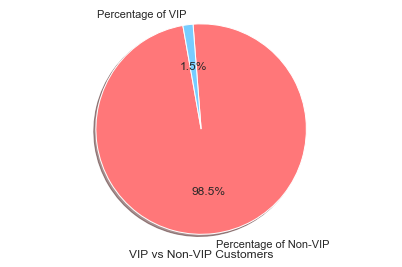Distribution of Majority and Minority Class

First few rows of the dataset

If we use this data for training our predictive model than the model will perform badly because the model is not trained on a sufficient amount of data representing VIP customers. Just to demonstrate how predictive models results will be if we use this data, below are the results of few algorithms: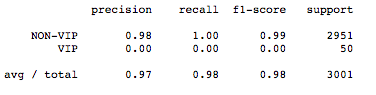Results from the k-NN Algorithm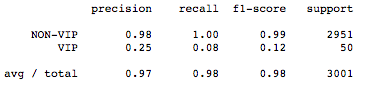Results from the Gradient Boosting Algorithm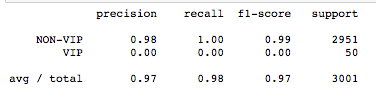Results from the Logistic Regression Algorithm

From the above results, it is very clear that the machine learning algorithms almost failed (because f1 score for VIP customer is very low)to predict the VIP customer.

In supervised learning, a common strategy to overcome the class imbalance problem is to resample the original training dataset to decrease the overall level of class imbalance. Resampling is done either by oversampling the minority (positive) class and/or under-sampling the majority (negative) class until the classes are approximately equally represented.

Before going to different re-sampling techniques one most important thing to keep in mind is that all resampling operations have to be applied to only training datasets. If upsampling is done before splitting the dataset into a train and validation set, then it could end up with the same observation in both datasets. As a result, a machine learning model will be able to perfectly predict the value for those observations when predicting on the validation set, hence inflating the accuracy and recall.

Types of re-sampling techniques:

Under/Down-sampling: This method uses a subset of the majority class to train the classifier. Since many majority class examples are ignored, the training set becomes more balanced and the training process becomes faster. The most common preprocessing technique is random majority under-sampling (RUS), In RUS, Instances of the majority class are randomly discarded from the dataset. Below is the python code for implementing downsampling.Oversampling: It achieves a more balanced class distribution by duplicating minority class instances. No information is lost in oversampling as all original instances of the minority and the majority classes are retained in the oversampled dataset. Below is the python code for implementing upsampling.Even though both approaches address the class imbalance problem, they also suffer some drawbacks. The random undersampling method can potentially remove certain important data points, and random oversampling can lead to overfitting.

SMOTE: Synthetic Minority Over-sampling Technique has been designed to generate new samples that are coherent with the minor class distribution. The main idea is to consider the relationships that exist between samples and create new synthetic points along the segments connecting a group of neighbors. Below is the python code for implementing SMOTE.Now our data is ready, let's apply some machine learning algorithms on the dataset created by SMOTE. I tried the following algorithms: Logistic Regression, K Nearest Neighbors, Gradient Boosting Classifier, Decision Tree, Random Forest, Neural Net. Below are the results and explanation of top performing machine learning algorithms :

Random Forest: The basic idea is very similar to bagging in the sense that we bootstrap samples, so we take a resample of our training data set. And then we rebuild classification or regression trees on each of those bootstrap samples. The one difference is that at each split when we split the data each time in a classification tree, we also bootstrap the variables. In other words, only a subset of the variables is considered at each potential split. This makes for a diverse set of potential trees that can be built. And so the idea is we grow a large number of trees. For prediction, we either vote or average those trees in order to get the prediction for a new outcome. Below is the python code for implementing Random Forest Classifier.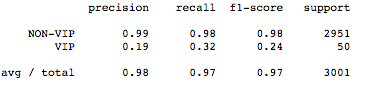The result of the Random Forest applied to dataset derived from SMOTE

Gradient Boosting: Unlike the random forest method that builds and combines a forest of randomly different trees in parallel, the key idea of gradient boosted decision trees is that they build a series of trees. Where each tree is trained so that it attempts to correct the mistakes of the previous tree in the series. Built in a non-random way, to create a model that makes fewer and fewer mistakes as more trees are added. Once the model is built, making predictions with a gradient boosted tree models is fast and doesn’t use a lot of memory. Below is the python code for implementing Gradient Boosting Classifier.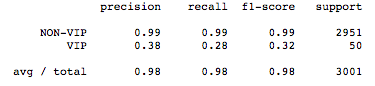The result of the Gradient Boosting applied to dataset derived from SMOTE

Ada Boost: It is an iterative ensemble method. AdaBoost classifier builds a strong classifier by combining multiple poorly performing classifiers to get high accuracy strong classifier. The basic concept behind Adaboost is to set the weights of classifiers and training the data sample in each iteration such that it ensures the accurate predictions of unusual observations. Below is the python code for implementing Ada Boost Classifier.The result of the Ada Boosting applied to dataset derived from SMOTE

Note: Predictive accuracy, a popular choice for evaluating the performance of a classifier, might not be appropriate when the data is imbalanced. It should not be used as it will not give a true picture. For example, the accuracy of the model might be 97% and one might think that model is performing extremely well but in reality, the model might be predicting only majority class and if the main purpose of the model is to predict minority class then model is of no use. Hence recall, precision and f1-score should be used for measuring the performance of the model.

Conclusion: So far we saw that by re-sampling imbalanced dataset and by choosing the right machine learning algorithm we can improve the prediction performance for minority class. Our best performing model was Ada and gradient boosting ran on new dataset synthesized using SMOTE. With these models, we achieved f1 score for minority class 0.32 while with raw data and with algorithms like logistic and k-nn, f1-score for minority class was 0.00

Further Improvements: To further improve the model, below options can be considered:

1. Try using variants of SMOTE.
2. Tuning of hyper-parameters(learning rate, max-depth, etc.) of the above models.
3. Also, different machine learning models like stacked or hybrid machine learning algorithms, deep learning models can be used.